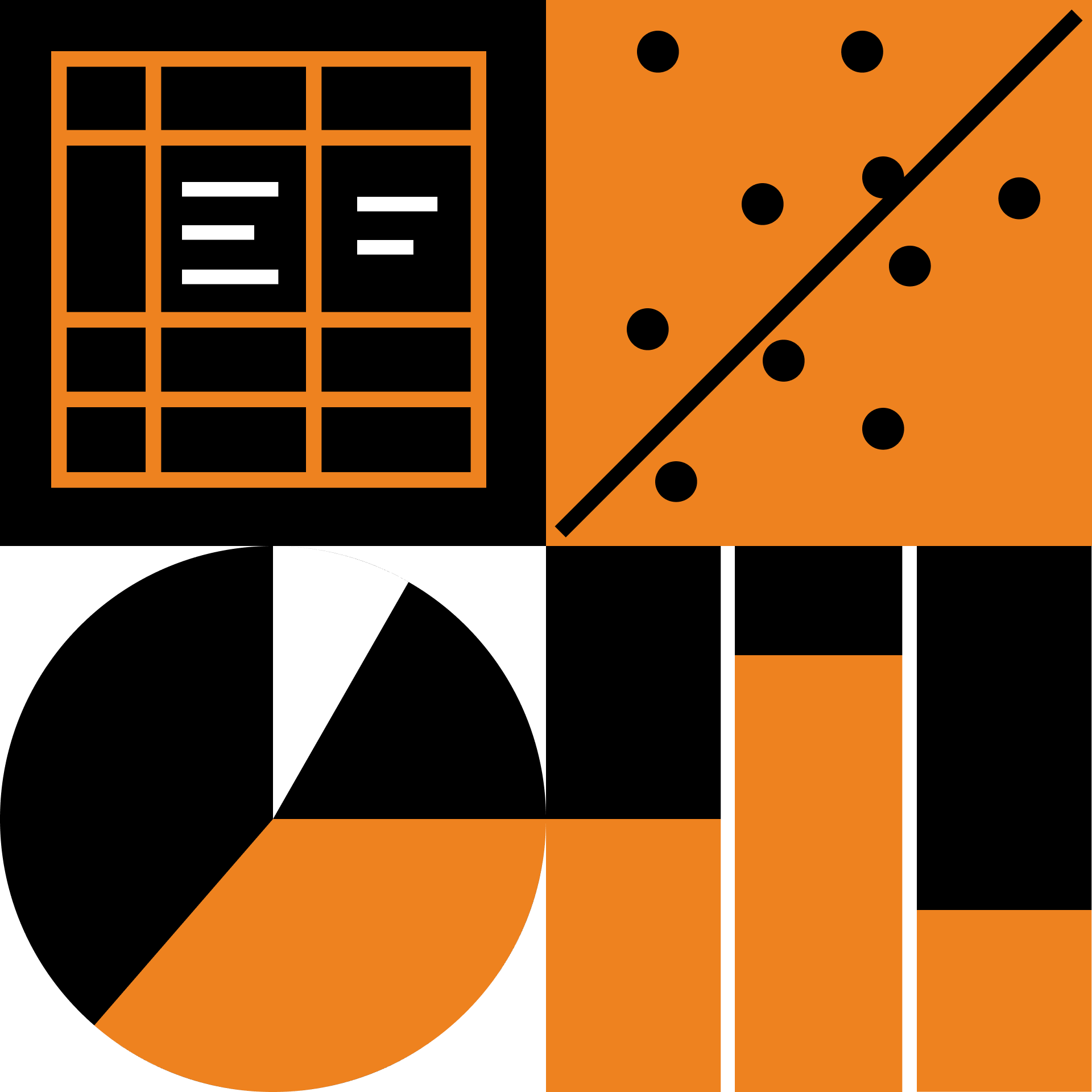Data Analysis · Level 4

## 4.2 Hypothesis Testing

Expand your statistics toolkit, learning how to make good decisions with limited data.

Hypothesis Testing

The Z-statistic

The Z-test

Understanding p-values

p-hacking

Power

Practice: Power & Error

Confidence Intervals

The Chi-Square Statistic I

The Chi-Square Statistic II

Chi-Square Random Variables

Degrees of Freedom I

Point Estimates

Degrees of Freedom II

Homogeneity Tests

Independence Tests

A Tale of Two Cities' Proportions

Intro to t-variables

Pooled Variance

Unpooled Variance

Why ANOVA?

Linear Regression: The Simplest Model

Best-Fit Lines

The Linear Regression F-statistic

Linear Regression ANOVA Tables

ANOVA and Mean Comparisons

### Course description

Have you ever wanted to use data to test a hypothesis, prove a point, or even just make meaning of the world? Statistics is essential for achieving all of those goals, and this course will teach you the methods you need to make the most of your data. You'll gain hands-on experience designing experiments and framing questions for statistical analysis. You'll also expand your statistics toolkit to include a suite of powerful hypothesis tests.

### Topics covered

• ANOVA
• Chi-square test
• Contingency tables
• F-test
• Goodness of fit
• Power
• p-value
• t-test

### Prerequisites and next steps

The basics of statistics covered in a first semester stats course is crucial. Familiarity with some basic concepts from probability, such as distribution functions, mean, variance, and the central limit theorem, is necessary. Some calculus knowledge will be helpful, but not essential.

Up next

Data Analysis · Level 5

### 5.1 Introduction to Neural Networks

Delve into the inner machinery of neural networks to discover how these flexible learning tools actually work.